# Tag: modular

Last time we revisited Robin’s theorem saying that 5040 being the largest counterexample to the bound
$\frac{\sigma(n)}{n~log(log(n))} < e^{\gamma} = 1.78107...$ is equivalent to the Riemann hypothesis.

There’s an industry of similar results using other arithmetic functions. Today, we’ll focus on Dedekind’s Psi function
$\Psi(n) = n \prod_{p | n}(1 + \frac{1}{p})$
where $p$ runs over the prime divisors of $n$. It is series A001615 in the online encyclopedia of integer sequences and it starts off with

1, 3, 4, 6, 6, 12, 8, 12, 12, 18, 12, 24, 14, 24, 24, 24, 18, 36, 20, 36, 32, 36, 24, 48, 30, 42, 36, 48, 30, 72, 32, 48, 48, 54, 48, …

and here’s a plot of its first 1000 values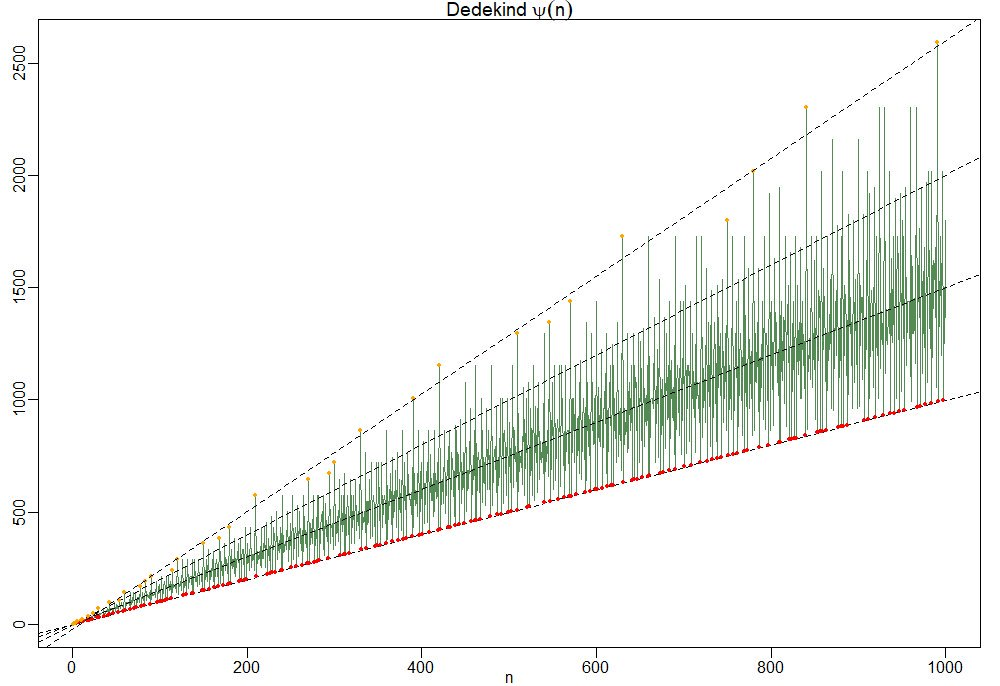To understand this behaviour it is best to focus on the ‘slopes’ $\frac{\Psi(n)}{n}=\prod_{p|n}(1+\frac{1}{p})$.

So, the red dots of minimal ‘slope’ $\approx 1$ correspond to the prime numbers, and the ‘outliers’ have a maximal number of distinct small prime divisors. Look at $210 = 2 \times 3 \times 5 \times 7$ and its multiples $420,630$ and $840$ in the picture.

For this reason the primorial numbers, which are the products of the fist $k$ prime numbers, play a special role. This is series A002110 starting off with

1, 2, 6, 30, 210, 2310, 30030, 510510, 9699690, 223092870,…

In Patrick Solé and Michel Planat Extreme values of the Dedekind $\Psi$ function, it is shown that the primorials play a similar role for Dedekind’s Psi as the superabundant numbers play for the sum-of-divisors function $\sigma(n)$.

That is, if $N_k$ is the $k$-th primorial, then for all $n < N_k$ we have that the 'slope' at $n$ is strictly below that of $N_k$ $\frac{\Psi(n)}{n} < \frac{\Psi(N_k)}{N_k}$ which follows immediately from the fact that any $n < N_k$ can have at most $k-1$ distinct prime factors and $p \mapsto 1 + \frac{1}{p}$ is a strictly decreasing function.

Another easy, but nice, observation is that for all $n$ we have the inequalities
$n^2 > \phi(n) \times \psi(n) > \frac{n^2}{\zeta(2)}$
where $\phi(n)$ is Euler’s totient function
$\phi(n) = n \prod_{p | n}(1 – \frac{1}{p})$
This follows as once from the definitions of $\phi(n)$ and $\Psi(n)$
$\phi(n) \times \Psi(n) = n^2 \prod_{p|n}(1 – \frac{1}{p^2}) < n^2 \prod_{p~\text{prime}} (1 - \frac{1}{p^2}) = \frac{n^2}{\zeta(2)}$ But now it starts getting interesting.

In the proof of his theorem, Guy Robin used a result of his Ph.D. advisor Jean-Louis Nicolasknown as Nicolas’ criterion for the Riemann hypothesis: RH is true if and only if for all $k$ we have the inequality for the $k$-th primorial number $N_k$
$\frac{N_k}{\phi(N_k)~log(log(N_k))} > e^{\gamma}$
From the above lower bound on $\phi(n) \times \Psi(n)$ we have for $n=N_k$ that
$\frac{\Psi(N_k)}{N_k} > \frac{N_k}{\phi(N_k) \zeta(2)}$
and combining this with Nicolas’ criterion we get
$\frac{\Psi(N_k)}{N_k~log(log(N_k))} > \frac{N_k}{\phi(N_k)~log(log(N_k)) \zeta(2)} > \frac{e^{\gamma}}{\zeta(2)} \approx 1.08…$
In fact, Patrick Solé and Michel Planat prove in their paper Extreme values of the Dedekind $\Psi$ function that RH is equivalent to the lower bound
$\frac{\Psi(N_k)}{N_k~log(log(N_k))} > \frac{e^{\gamma}}{\zeta(2)}$
holding for all $k \geq 3$.

Dedekind’s Psi function pops up in lots of interesting mathematics.

In the theory of modular forms, Dedekind himself used it to describe the index of the congruence subgroup $\Gamma_0(n)$ in the full modular group $\Gamma$.

In other words, it gives us the number of tiles needed in the Dedekind tessellation to describe the fundamental domain of the action of $\Gamma_0(n)$ on the upper half-plane by Moebius transformations.When $n=6$ we have $\Psi(6)=12$ and we can view its fundamental domain via these Sage commands:

 G=Gamma0(6) FareySymbol(G).fundamental_domain() 

giving us the 24 back or white tiles (note that these tiles are each fundamental domains of the extended modular group, so we have twice as many of them as for subgroups of the modular group)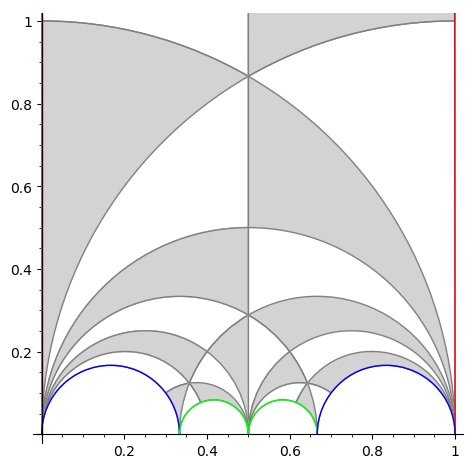But, there are plenty of other, seemingly unrelated, topics where $\Psi(n)$ appears. To name just a few:

• The number of points on the projective line $\mathbb{P}^1(\mathbb{Z}/n\mathbb{Z})$.
• The number of lattices at hyperdistance $n$ in Conway’s big picture.
• The number of admissible maximal commuting sets of operators in the Pauli group for the $n$ qudit.

and there are explicit natural one-to-one correspondences between all these manifestations of $\Psi(n)$, tbc.

I’ve LaTeXed $48=2 \times 24$ posts into a 114 page booklet Monsters and Moonshine for you to download.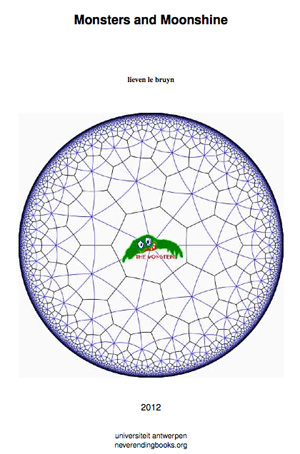The $24$ ‘Monsters’ posts are (mostly) about finite simple (sporadic) groups : we start with the Scottish solids (hoax?), move on to the 14-15 game groupoid and a new Conway $M_{13}$-sliding game which uses the sporadic Mathieu group $M_{12}$. This Mathieu group appears in musical compositions of Olivier Messiaen and it can be used also to get a winning strategy of ‘mathematical blackjack’. We discuss Galois’ last letter and the simple groups $L_2(5),L_2(7)$ and $L_2(11)$ as well as other Arnold ‘trinities’. We relate these groups to the Klein quartic and the newly discovered ‘buckyball’-curve. Next we investigate the history of the Leech lattice and link to online games based on the Mathieu-groups and Conway’s dotto group. Finally, preparing for moonshine, we discover what the largest sporadic simple group, the Monster-group, sees of the modular group.

The $24$ ‘Moonshine’ posts begin with the history of the Dedekind (or Klein?) tessellation of the upper half plane, useful to determine fundamental domains of subgroups of the modular group $PSL_2(\mathbb{Z})$. We investigate Grothendieck’s theory of ‘dessins d’enfants’ and learn how modular quilts classify the finite index subgroups of the modular group. We find generators of such groups using Farey codes and use those to give a series of simple groups including as special members $L_2(5)$ and the Mathieu-sporadics $M_{12}$ and $M_{24}$ : the ‘iguanodon’-groups. Then we move to McKay-Thompson series and an Easter-day joke pulled by John McKay. Apart from the ‘usual’ monstrous moonshine conjectures (proved by Borcherds) John McKay also observed a strange appearance of $E(8)$ in connection with multiplications of involutions in the Monster-group. We explain Conway’s ‘big picture’ which makes it easy to work with the moonshine groups and use it to describe John Duncan’s solution of the $E(8)$-observation.

I’ll try to improve the internal referencing over the coming weeks/months, include an index and add extra material as we will be studying moonshine for the Mathieu groups as well as a construction of the Monster-group in next semester’s master-seminar. All comments, corrections and suggestions for extra posts are welcome!

If you are interested you can also download two other booklets : The Bourbaki Code (38 pages) containing all Bourbaki-related posts and absolute geometry (63 pages) containing the posts related to the “field with one element” and its connections to (noncommutative) geometry and number theory.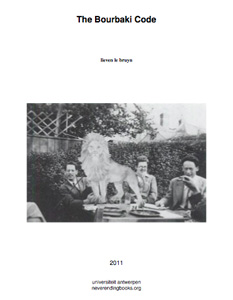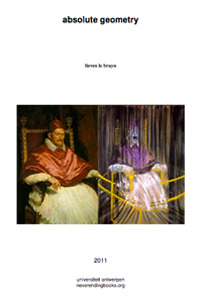I’ll try to add to the ‘absolute geometry’-booklet the posts from last semester’s master-seminar (which were originally posted at angs@t/angs+) and write some new posts covering the material that so far only exists as prep-notes. The links above will always link to the latest versions of these booklets.

Sunday january 2nd around 18hr NeB-stats went crazy.

Referrals clarified that the post ‘What is the knot associated to a prime?’ was picked up at Reddit/math and remained nr.1 for about a day.

Now, the dust has settled, so let’s learn from the experience.A Reddit-mention is to a blog what doping is to a sporter.

You get an immediate boost in the most competitive of all blog-stats, the number of unique vistors (blue graph), but is doesn’t result in a long-term effect, and, it may even be harmful to more essential blog-stats, such as the average time visitors spend on your site (yellow graph).

For NeB the unique vistors/day fluctuate normally around 300, but peaked to 1295 and 1733 on the ‘Reddit-days’. In contrast, the avg. time on site is normally around 3 minutes, but dropped the same days to 44 and 30 seconds!

Whereas some of the Reddits spend enough time to read the post and comment on it, the vast majority zap from one link to the next. Having monitored the Reddit/math page for two weeks, I’m convinced that post only made it because it was visually pretty good. The average Reddit/math-er is a viewer more than a reader…

So, should I go for shorter, snappier, more visual posts?

Let’s compare Reddits to those coming from the three sites giving NeB most referrals : Google search, MathOverflow and Wikipedia.This is the traffic coming from Reddit/math, as always the blue graph are the unique visitors, the yellow graph their average time on site, blue-scales to the left, yellow-scales to the right.

Here’s the same graph for Google search. The unique visitors/day fluctuate around 50 and their average time on site about 2 minutes.The math-related search terms most used were this month : ‘functor of point approach’, ‘profinite integers’ and ‘bost-connes sytem’.

More rewarding to me are referrals from MathOverflow.The number of visitors depends on whether the MathO-questions made it to the front-page (for example, the 80 visits on december 15, came from the What are dessins d’enfants?-topic getting an extra comment that very day, and having two references to NeB-posts : The best rejected proposal ever and Klein’s dessins d’enfant and the buckyball), but even older MathO-topics give a few referrals a day, and these people sure take their time reading the posts (+ 5 minutes).

Other MathO-topics giving referrals this month were Most intricate and most beautiful structures in mathematics (linking to Looking for F-un), What should be learned in a first serious schemes course? (linking to Mumford’s treasure map (btw. one of the most visited NeB-posts ever)), How much of scheme theory can you visualize? (linking again to Mumford’s treasure map) and Approaches to Riemann hypothesis using methods outside number theory (linking to the Bost-Connes series).

Finally, there’s Wikipediagiving 5 to 10 referrals a day, with a pretty good time-on-site average (around 4 minutes, peaking to 12 minutes). It is rewarding to see NeB-posts referred to in as diverse Wikipedia-topics as ‘Fifteen puzzle’, ‘Field with one element’, ‘Evariste Galois’, ‘ADE classification’, ‘Monster group’, ‘Arithmetic topology’, ‘Dessin d’enfant’, ‘Groupoid’, ‘Belyi’s theorem’, ‘Modular group’, ‘Cubic surface’, ‘Esquisse d’un programme’, ‘N-puzzle’, ‘Shabat polynomial’ and ‘Mathieu group’.

What lesson should be learned from all this data? Should I go for shorter, snappier and more visual posts, or should I focus on the small group of visitors taking their time reading through a longer post, and don’t care about the appallingly high bounce rate the others cause?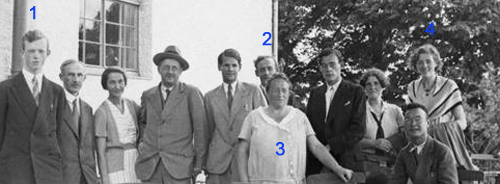For the better part of the 30ties, Ernst Witt (1) did hang out with the rest of the ‘Noetherknaben’, the group of young mathematicians around Emmy Noether (3) in Gottingen.

In 1934 Witt became Helmut Hasse‘s assistent in Gottingen, where he qualified as a university lecturer in 1936. By 1938 he has made enough of a name for himself to be offered a lecturer position in Hamburg and soon became an associate professor, the down-graded position held by Emil Artin (2) until he was forced to emigrate in 1937.

A former fellow student of him in Gottingen, Erna Bannow (4), had gone earlier to Hamburg to work with Artin. She continued her studies with Witt and finished her Ph.D. in 1939. In 1940 Erna Bannow and Witt married.

So, life was smiling on Ernst Witt that sunday january 28th 1940, both professionally and personally. There was just one cloud on the horizon, and a rather menacing one. He was called up by the Wehrmacht and knew he had to enter service in february. For all he knew, he was spending the last week-end with his future wife… (later in february 1940, Blaschke helped him to defer his military service by one year).

Still, he desperately wanted to finish his paper before entering the army, so he spend most of that week-end going through the final version and submitted it on monday, as the published paper shows.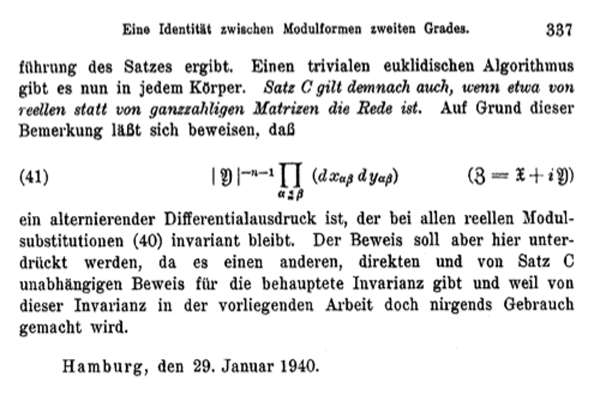In the 70ties, Witt suddenly claimed he did discover the Leech lattice ${\Lambda}$ that sunday. Last time we have seen that the only written evidence for Witt’s claim is one sentence in his 1941-paper Eine Identität zwischen Modulformen zweiten Grades. “Bei dem Versuch, eine Form aus einer solchen Klassen wirklich anzugeben, fand ich mehr als 10 verschiedene Klassen in ${\Gamma_{24}}$.”

But then, why didn’t Witt include more details of this sensational lattice in his paper?

Ina Kersten recalls on page 328 of Witt’s collected papers : “In his colloquium talk “Gitter und Mathieu-Gruppen” in Hamburg on January 27, 1970, Witt said that in 1938, he had found nine lattices in ${\Gamma_{24}}$ and that later on January 28, 1940, while studying the Steiner system ${S(5,8,24)}$, he had found two additional lattices ${M}$ and ${\Lambda}$ in ${\Gamma_{24}}$. He continued saying that he had then given up the tedious investigation of ${\Gamma_{24}}$ because of the surprisingly low contribution

$\displaystyle | Aut(\Lambda) |^{-1} < 10^{-18}$

to the Minkowski density and that he had consented himself with a short note on page 324 in his 1941 paper.”

In the last sentence he refers to the fact that the sum of the inverse orders of the automorphism groups of all even unimodular lattices of a given dimension is a fixed rational number, the Minkowski-Siegel mass constant. In dimension 24 this constant is

$\displaystyle \sum_{L} \frac{1}{| Aut(L) |} = \frac {1027637932586061520960267}{129477933340026851560636148613120000000} \approx 7.937 \times 10^{-15}$

That is, Witt was disappointed by the low contribution of the Leech lattice to the total constant and concluded that there might be thousands of new even 24-dimensional unimodular lattices out there, and dropped the problem.

If true, the story gets even better : not only claims Witt to have found the lattices ${A_1^{24}=M}$ and ${\Lambda}$, but also enough information on the Leech lattice in order to compute the order of its automorphism group ${Aut(\Lambda)}$, aka the Conway group ${Co_0 = .0}$ the dotto-group!

Is this possible? Well fortunately, the difficulties one encounters when trying to compute the order of the automorphism group of the Leech lattice from scratch, is one of the better documented mathematical stories around.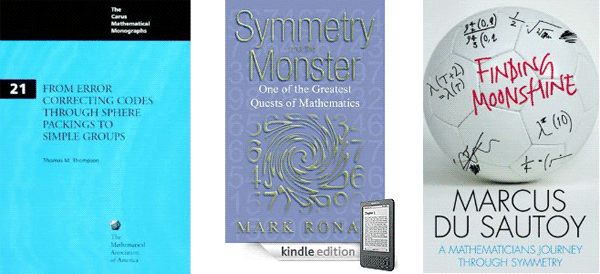The books From Error-Correcting Codes through Sphere Packings to Simple Groups by Thomas Thompson, Symmetry and the monster by Mark Ronan, and Finding moonshine by Marcus du Sautoy tell the story in minute detail.

It took John Conway 12 hours on a 1968 saturday in Cambridge to compute the order of the dotto group, using the knowledge of Leech and McKay on the properties of the Leech lattice and with considerable help offered by John Thompson via telephone.

But then, John Conway is one of the fastest mathematicians the world has known. The prologue of his book On numbers and games begins with : “Just over a quarter of a century ago, for seven consecutive days I sat down and typed from 8:30 am until midnight, with just an hour for lunch, and ever since have described this book as “having been written in a week”.”

Conway may have written a book in one week, Ernst Witt did complete his entire Ph.D. in just one week! In a letter of August 1933, his sister told her parents : “He did not have a thesis topic until July 1, and the thesis was to be submitted by July 7. He did not want to have a topic assigned to him, and when he finally had the idea, he started working day and night, and eventually managed to finish in time.”

So, if someone might have beaten John Conway in fast-computing the dottos order, it may very well have been Witt. Sadly enough, there is a lot of circumstantial evidence to make Witt’s claim highly unlikely.

For starters, psychology. Would you spend your last week-end together with your wife to be before going to war performing an horrendous calculation?

Secondly, mathematical breakthroughs often arise from newly found insight. At that time, Witt was also working on his paper on root lattices “Spiegelungsgrupen and Aufzähling halbeinfacher Liescher Ringe” which he eventually submitted in january 1941. Contained in that paper is what we know as Witt’s lemma which tells us that for any integral lattice the sublattice generated by vectors of norms 1 and 2 is a direct sum of root lattices.

This leads to the trick of trying to construct unimodular lattices by starting with a direct sum of root lattices and ‘adding glue’. Although this gluing-method was introduced by Kneser as late as 1967, Witt must have been aware of it as his 16-dimensional lattice ${D_{16}^+}$ is constructed this way.

If Witt wanted to construct new 24-dimensional even unimodular lattices in 1940, it would be natural for him to start off with direct sums of root lattices and trying to add vectors to them until he got what he was after. Now, all of the Niemeier-lattices are constructed this way, except for the Leech lattice!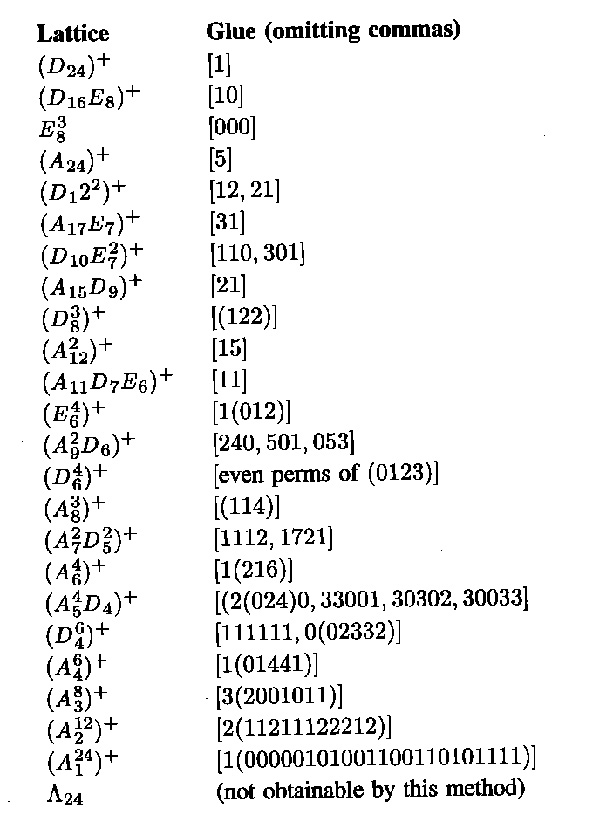I’m far from an expert on the Niemeier lattices but I would say that Witt definitely knew of the existence of ${D_{24}^+}$, ${E_8^3}$ and ${A_{24}^+}$ and that it is quite likely he also constructed ${(D_{16}E_8)^+, (D_{12}^2)^+, (A_{12}^2)^+, (D_8^3)^+}$ and possibly ${(A_{17}E_7)^+}$ and ${(A_{15}D_9)^+}$. I’d rate it far more likely Witt constructed another two such lattices on sunday january 28th 1940, rather than discovering the Leech lattice.

Finally, wouldn’t it be natural for him to include a remark, in his 1941 paper on root lattices, that not every even unimodular lattices can be obtained from sums of root lattices by adding glue, the Leech lattice being the minimal counter-example?

If it is true he was playing around with the Steiner systems that sunday, it would still be a pretty good story he discovered the lattices ${(A_2^{12})^+}$ and ${(A_1^{24})^+}$, for this would mean he discovered the Golay codes in the process!

Which brings us to our next question : who discovered the Golay code?

The Leech lattice was, according to wikipedia, ‘originally discovered by Ernst Witt in 1940, but he did not publish his discovery’ and it ‘was later re-discovered in 1965 by John Leech’. However, there is very little evidence to support this claim.

The facts

What is certain is that John Leech discovered in 1965 an amazingly dense 24-dimensional lattice ${\Lambda}$ having the property that unit balls around the lattice points touch, each one of them having exactly 196560 neighbors. The paper ‘Notes on sphere packings’ appeared in 1967 in the Canad. J. Math. 19, 251-267.

Compare this to the optimal method to place pennies on a table, leading to the hexagonal tiling, each penny touching exactly 6 others. Similarly, in dimension 8 the densest packing is the E8 lattice in which every unit ball has exactly 240 neighbors.

The Leech lattice ${\Lambda}$ can be characterized as the unique unimodular positive definite even lattice such that the length of any non-zero vector is at least two.

The list of all positive definite even unimodular lattices, ${\Gamma_{24}}$, in dimension 24 was classified later by Hans-Volker Niemeier and are now known as the 24 Niemeier lattices.For the chronology below it is perhaps useful to note that, whereas Niemeier’s paper did appear in 1973, it was submitted april 5th 1971 and is just a minor rewrite of Niemeier’s Ph.D. “Definite quadratische Formen der Dimension 24 und Diskriminante 1” obtained in 1968 from the University of Göttingen with advisor Martin Kneser.

The claim

On page 328 of Ernst Witt’s Collected Papers Ina Kersten recalls that Witt gave a colloquium talk on January 27, 1970 in Hamburg entitled “Gitter und Mathieu-Gruppen” (Lattices and Mathieu-groups). In this talk Witt claimed to have found nine lattices in ${\Gamma_{24}}$ as far back as 1938 and that on January 28, 1940 he found two additional lattices ${M}$ and ${\Lambda}$ while studying the Steiner system ${S(5,8,24)}$.

On page 329 of the collected papers is a scan of the abstract Witt wrote in the colloquium book in Bielefeld where he gave a talk “Uber einige unimodularen Gitter” (On certain unimodular lattices) on January 28, 1972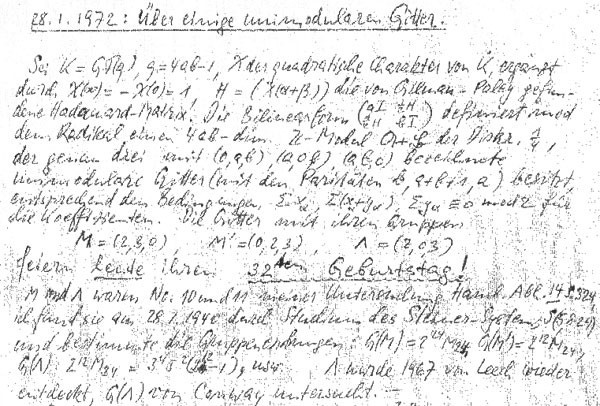Here, Witt claims that he found three new lattices in ${\Gamma_{24}}$ on January 28, 1940 as the lattices ${M}$, ${M’}$ and ${\Lambda}$ ‘feiern heute ihren 32sten Gebursttag!’ (celebrate today their 32nd birthday).

He goes on telling that the lattices ${M}$ and ${\Lambda}$ were number 10 and 11 in his list of lattices in ${\Gamma_{24}}$ in his paper “Eine Identität zwischen Modulformen zweiten Grades” in the Abh. Math. Sem. Univ. Hamburg 14 (1941) 323-337 and he refers in particular to page 324 of that paper.

He further claims that he computed the orders of their automorphism groups and writes that ${\Lambda}$ ‘wurde 1967 von Leech wieder-entdeckt’ (was re-discovered by Leech in 1967) and that its automorphism group ${G(\Lambda)}$ was studied by John Conway. Recall that Conway’s investigations of the automorphism group of the Leech lattice led to the discovery of three new sporadic groups, the Conway groups ${Co_1,Co_2}$ and ${Co_3}$.

However, Witt’s 1941-paper does not contain a numbered list of 24-dimensional lattices. In fact, apart from ${E_8+E_8+E_8}$ is does not contain a single lattice in ${\Gamma_{24}}$. The only relevant paragraph is indeed on page 324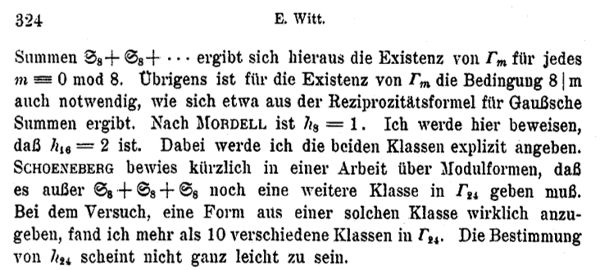He observes that Mordell already proved that there is just one lattice in ${\Gamma_8}$ (the ${E_8}$-lattice) and that the main result of his paper is to prove that there are precisely two even unimodular 16-dimensional lattices : ${E_8+E_8}$ and another lattice, now usually called the 16-dimensional Witt-lattice.

He then goes on to observe that Schoeneberg knew that ${\# \Gamma_{24} > 1}$ and so there must be more lattices than ${E_8+E_8+E_8}$ in ${\Gamma_{24}}$. Witt concludes with : “In my attempt to find such a lattice, I discovered more than 10 lattices in ${\Gamma_{24}}$. The determination of ${\# \Gamma_{24}}$ does not seem to be entirely trivial.”

Hence, it is fair to assume that by 1940 Ernst Witt had discovered at least 11 of the 24 Niemeier lattices. Whether the Leech lattice was indeed lattice 11 on the list is anybody’s guess.

Next time we will look more closely into the historical context of Witt’s 1941 paper.

This is a belated response to a Math-Overflow exchange between Thomas Riepe and Chandan Singh Dalawat asking for a possible connection between Connes’ noncommutative geometry approach to the Riemann hypothesis and the Langlands program.

Here’s the punchline : a large chunk of the Connes-Marcolli book Noncommutative Geometry, Quantum Fields and Motives can be read as an exploration of the noncommutative boundary to the Langlands program (at least for $GL_1$ and $GL_2$ over the rationals $\mathbb{Q}$).

Recall that Langlands for $GL_1$ over the rationals is the correspondence, given by the Artin reciprocity law, between on the one hand the abelianized absolute Galois group

$Gal(\overline{\mathbb{Q}}/\mathbb{Q})^{ab} = Gal(\mathbb{Q}(\mu_{\infty})/\mathbb{Q}) \simeq \hat{\mathbb{Z}}^*$

and on the other hand the connected components of the idele classes

$\mathbb{A}^{\ast}_{\mathbb{Q}}/\mathbb{Q}^{\ast} = \mathbb{R}^{\ast}_{+} \times \hat{\mathbb{Z}}^{\ast}$

The locally compact Abelian group of idele classes can be viewed as the nice locus of the horrible quotient space of adele classes $\mathbb{A}_{\mathbb{Q}}/\mathbb{Q}^{\ast}$. There is a well-defined map

$\mathbb{A}_{\mathbb{Q}}’/\mathbb{Q}^{\ast} \rightarrow \mathbb{R}_{+} \qquad (x_{\infty},x_2,x_3,\ldots) \mapsto | x_{\infty} | \prod | x_p |_p$

from the subset $\mathbb{A}_{\mathbb{Q}}’$ consisting of adeles of which almost all terms belong to $\mathbb{Z}_p^{\ast}$. The inverse image of this map over $\mathbb{R}_+^{\ast}$ are precisely the idele classes $\mathbb{A}^{\ast}_{\mathbb{Q}}/\mathbb{Q}^{\ast}$. In this way one can view the adele classes as a closure, or ‘compactification’, of the idele classes.

This is somewhat reminiscent of extending the nice action of the modular group on the upper-half plane to its badly behaved action on the boundary as in the Manin-Marcolli cave post.

The topological properties of the fiber over zero, and indeed of the total space of adele classes, are horrible in the sense that the discrete group $\mathbb{Q}^*$ acts ergodically on it, due to the irrationality of $log(p_1)/log(p_2)$ for primes $p_i$. All this is explained well (in the semi-local case, that is using $\mathbb{A}_Q’$ above) in the Connes-Marcolli book (section 2.7).

In much the same spirit as non-free actions of reductive groups on algebraic varieties are best handled using stacks, such ergodic actions are best handled by the tools of noncommutative geometry. That is, one tries to get at the geometry of $\mathbb{A}_{\mathbb{Q}}/\mathbb{Q}^{\ast}$ by studying an associated non-commutative algebra, the skew-ring extension of the group-ring of the adeles by the action of $\mathbb{Q}^*$ on it. This algebra is known to be Morita equivalent to the Bost-Connes algebra which is the algebra featuring in Connes’ approach to the Riemann hypothesis.

It shouldn’t thus come as a major surprise that one is able to recover the other side of the Langlands correspondence, that is the Galois group $Gal(\mathbb{Q}(\mu_{\infty})/\mathbb{Q})$, from the Bost-Connes algebra as the symmetries of certain states.

In a similar vein one can read the Connes-Marcolli $GL_2$-system (section 3.7 of their book) as an exploration of the noncommutative closure of the Langlands-space $GL_2(\mathbb{A}_{\mathbb{Q}})/GL_2(\mathbb{Q})$.

At the moment I’m running a master-seminar noncommutative geometry trying to explain this connection in detail. But, we’re still in the early phases, struggling with the topology of ideles and adeles, reciprocity laws, L-functions and the lot. Still, if someone is interested I might attempt to post some lecture notes here.

A few days before Halloween, Norbert Dufourcq (who died december 17th 1990…), sent me a comment, containing lots of useful information, hinting I did get it wrong about the church of the Bourbali wedding in the previous post.

Norbert Dufourcq, an organist and student of Andre Machall, the organist-in-charge at the Saint-Germain-des-Prés church in 1939, the place where I speculated the Bourbaki wedding took place, concluded his comment with :

“P.S. Lieven, you _do_ know about the Schola Cantorum, now, don’t you?!?”.

Euh… actually … no, I did not …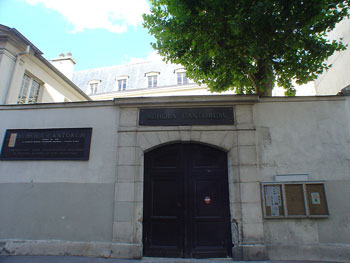La Schola Cantorum is a private music school in Paris. It was founded in 1894 by Charles Bordes, Alexandre Guilmant and Vincent d’Indy as a counterbalance to the Paris Conservatoire’s emphasis on opera. Its alumni include many significant figures in 20th century music, such as Erik Satie and Cole Porter.

Schola Cantorum is situated 69, rue Saint Jacques, Paris, just around the corner of the Ecole Normal Superieure, home base to the Bourbakis. In fact, closer investigation reveals striking similarities and very close connections between the circle of artists at la Schola and the Bourbaki group.

In december 1934, the exact month the Bourbaki group was formed, a radical reorganisation took place at the Schola, when Nestor Lejeune became the new director. He invited several young musicians, many from the famous Dukas-class, to take up teaching positions at the Schola.

Here’s a picture of part of the Dukas class of 1929, several of its members will play a role in the upcoming events :
from left to right next to the piano : Pierre Maillard-Verger, Elsa Barraine, Yvonne Desportes, Tony Aubin, Pierre Revel, Georges Favre, Paul Dukas, René Duclos, Georges Hugon, Maurice Duruflé. Seated on the right : Claude Arrieu, Olivier Messiaen.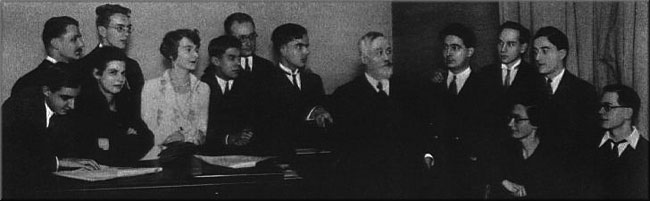The mid-1930s in Paris saw the emergence of two closely-related groups with a membership which overlapped : La Spirale and La Jeune France. La Spirale was founded in 1935 under the leadership of Georges Migot; its other committee members were Paul Le Flem, his pupil André Jolivet, Edouard Sciortino, Claire Delbos, her husband Olivier Messiaen, Daniel-Lesur and Jules Le Febvre. The common link between almost all of these musicians was their connection with the Schola Cantorum.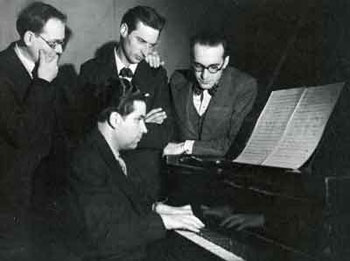On the left : Les Jeunes Musiciens Français : André Jolivet on the Piano. Standing from left to right :
Olivier Messiaen, Yves Baudrier, Daniel-Lesur.

Nigel Simeone wrote this about Messiaen and La Jeune France :
“The extremely original and independent-minded Messiaen had already shown himself to be a rather unexpected enthusiast for joining groups: in December 1932 he wrote to his friend Claude Arrieu about a letter from another musician, Jacques Porte, outlining plans for a new society to be called Les Jeunes Musiciens Français.
Messiaen agreed to become its vice-president, but nothing seems to have come of the project. Six months later, in June 1933, he had a frustrating meeting with Roger Désormière on behalf of the composers he described to Arrieu as ‘les quatre’, all of them Dukas pupils: Elsa Barraine, the recently-deceased Jean Cartan, Arrieu and Messiaen himself; during the early 1930s Messiaen and Arrieu organised concerts featuring all four composers.”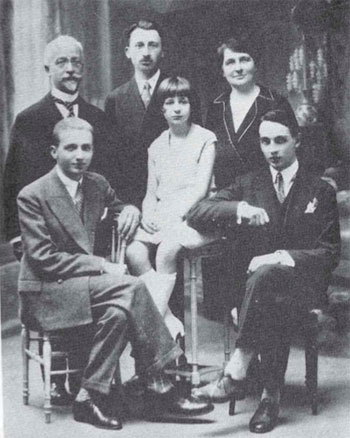Finally, we’re getting a connection with the Bourbaki group! Norbert Dufourcq mentioned it already in his comment “Messiaen was also a good friend of Jean Cartan (himself a composer, and Henri’s brother)”. Henri Cartan was one of the first Bourbakis and an excellent piano player himself.

The Cartan family picture on the right : standing from left to right, father Elie Cartan (one of the few older French mathematicians respected by the Bourbakis), Henri and his mother Marie-Louise. Seated, the younger children, from left to right : Louis, Helene (who later became a mathematician, herself) and the composer Jean Cartan, who sadly died very young from tuberculoses in 1932…

The december 1934 revolution in French music at the Schola Cantorum, instigated by Messiaen and followers, was the culmination of a process that started a few years before when Jean Cartan was among the circle of revolutionados. Because Messiaen was a fiend of the Cartan family, they surely must have been aware of the events at the Schola (or because it was merely a block away from the ENS), and, the musicians’ revolt may very well have been an example to follow for the first Bourbakis…(?!)

Anyway, we now know the intended meaning of the line “with lemmas sung by the Scholia Cartanorum” on the wedding-invitation. Cartanorum is NOT (as I claimed last time) bad Latin for ‘Cartesiorum’, leading to Descartes and the Saint-Germain-des-Pres church, but is in fact passable Latin (plur. gen.) of CARTAN(us), whence the translation “with lemmas sung by the school of the Cartans”. There’s possibly a double pun intended here : first, a reference to (father) Cartan’s lemma and, of course, to La Schola where the musical Cartan-family felt at home.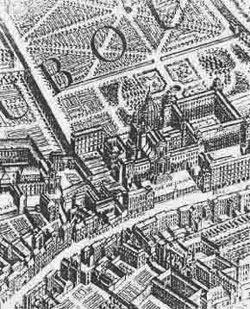Fine, but does this brings us any closer to the intended place of the Bourbaki-Petard wedding? Well, let’s reconsider the hidden ‘clues’ we discovered last time : the phrase “They will receive the trivial isomorphism from P. Adic, of the Order of the Diophantines” might suggest that the church belongs to a a religious order and is perhaps an abbey- or convent-church and the phrase “the organ will be played by Monsieur Modulo” requires us to identify this mysterious Mister Modulo, because Norbert Dufourcq rightfully observed :

“note however that in 1939, it wasn’t as common to have a friend-organist perform at a wedding as it is today: the appointed organists, especially at prestigious Paris positions, were much less likely to accept someone play in their stead.”

The history of La Schola Cantorum reveals something that might have amused Frank Smithies (remember he was one of the wedding-invitation-composers) : the Schola is located in the Convent(!) of the Brittish Benedictines…

In 1640 some Benedictine monks, on the run after the religious schism in Britain, found safety in Paris under the protection of Cardinal Richelieu and Anne of Austria at Val-de-Grace, where the Schola is now housed.

As is the case with most convents, the convent of the Brittish Benedictines did have its own convent church, now called l’église royale Notre-Dame du Val-de-Grâce (remember that one of the possible interpretations for “of the universal variety” was that the name of the church would be “Notre-Dame”…).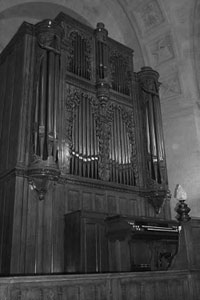This church is presently used as the concert hall of La Schola and is famous for its … musical organ : “In 1853, Aristide Cavaillé-Coll installed a new organ in the Church of Sainte-geneviève which had been restored in its rôle as a place of worship by Prince President Louis-Napoléon. In 1885, upon the decision of President Jules Grévy, this church once again became the Pantheon and, six years later, according to an understanding between the War and Public Works Departments, the organ was transferred to the Val-de-Grâce, under the supervision of the organ builder Merklin. Beforehand, the last time it was heard in the Pantheon must have been for the funeral service of Victor Hugo.
In 1927, a raising was carried out by the builder Paul-Marie Koenig, and the inaugural concert was given by André Marchal and Achille Philippe, the church’s organist. Added to the register of historic monument in 1979, Val-de-Grâce’s “ little great organ ”, as Cavaillé-Coll called it, was restored in 1993 by the organ builders François Delangue and Bernard Hurvy.
The organ of Val-de-Grâce is one the rare parisian surviving witnesses of the art of Aristide Cavaillé-Coll, an instrument that escaped abusive and definitive transformations or modernizations. This explain why, in spite of its relatively modest scale, this organ enjoys quite a reputation, and this, as far as the United States.”

By why would the Val-de-Grace organiste at the time Achille Philip, “organiste titulaire du Val-de-Grâce de 1903 à 1950 et professeur d’orgue et d’harmonie à la Schola Cantorum de 1904 à 1950”, be called ‘Mister Modulo’ in the wedding-invitations line “L’orgue sera tenu par Monsieur Modulo”???

Again, the late Norbert Dufourcq comes to our rescue, proposing a good candidate for ‘Monsieur Modulo’ : “As for “modulo”, note that the organist at Notre-Dame at that time, Léonce de Saint-Martin, was also the composer of a “Suite Cyclique”, though I admit that this is just wordplay: there is nothing “modular” about this work. Maybe a more serious candidate would be Olivier Messiaen (who was organist at the Église de la Trinité): his “modes à transposition limitée” are really about Z/12Z→Z/3Z and Z/12Z→Z/4Z. “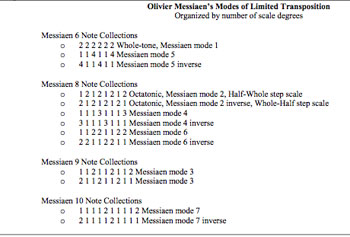Messiaen’s ‘Modes of limited transposition’ were compiled in his book ‘Technique de mon langage musical’. This book was published in Paris by Leduc, as late as 1944, 5 years after the wedding-invitation.

Still, several earlier works of Messiaen used these schemes, most notably La Nativité du Seigneur, composed in 1935 : “The work is one of the earliest to feature elements that were to become key to Messiaen’s later compositions, such as the extensive use of the composer’s own modes of limited transposition, as well as influence from birdsong, and the meters and rhythms of Ancient Greek and traditional Indian music.”

More details on Messiaen’s modes and their connection to modular arithmetic can be found in the study Implementing Modality in Algorithmic Composition by Vincent Joseph Manzo.

Hence, Messiaen is a suitable candidate for the title ‘Monsieur Modulo’, but would he be able to play the Val-de-Grace organ while not being the resident organist?

Remember, the Val-de-Grace church was the concert hall of La Schola, and its musical organ the instrument of choice for the relevant courses. Now … Olivier Messiaen taught at the Schola Cantorum and the École Normale de Musique from 1936 till 1939. So, at the time of the Bourbaki-Petard wedding he would certainly be allowed to play the Cavaillé-Coll organ.

Perhaps we got it right, the second time around : the Bourbaki-Pétard wedding was held on June 3rd 1939 in the church ‘l’église royale Notre-Dame du Val-de-Grâce’ at 12h?

Probably the smartest move I’ve made after entering math-school was to fall in love with a feminist.

Yeah well, perhaps I’ll expand a bit on this sentence another time. For now, suffice it to say that I did pick up a few words in the process, among them : the queen bee syndrome :

women who have attained senior positions do not use their power to assist struggling young women or to change the system, thereby tacitly validating it.

A recent study by the Max Planck Institute for Human Development asserts that the QBS

likely stems from women at the top who feel threatened by other women and therefore, prefer to surround themselves with men. As a result, these Queen Bees often jeapordize the promotions of other females at their companies.

Radical feminists of the late 70-ties preferred a different ‘explanation’, clearly.

Women who fought their way to the top, they said, were convinced that overcoming all obstacles along the way made them into the strong persons they became. A variant on the ‘what doesn’t kill you, makes you stronger’-mantra, quoi. These queen bees genuinely believed it to be beneficial to the next generation of young women not to offer them any shortcuts on their journey through the glass ceiling.

By and large, the 45+generation decides about the topics that should be (or shouldn’t be) on the current math-curriculum. They also write most of the text-books and course-notes used, and inevitably, the choices they make have an impact on the new generation of math-students.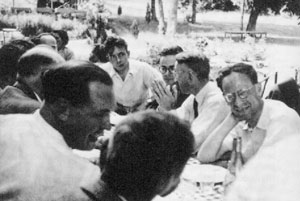Perhaps too little thought is given to the fact that the choices we (yes, I belong to that age group) make, the topics we deem important for new students to master, are heavily influenced by our own experiences.

In the late 60ties, early 70ties, Bourbaki-style mathematics influenced the ‘modern mathematics’ revolution in schools, certainly in Belgium through the influence of George Papy.

In kintergarten, kids learned the basics of set theory. Utensils to draw Venn diagrams were as indispensable as are pocket-calculators today. In secondary school, we had a formal axiomatic approach to geometry, we learned abstract topological spaces and other advanced topics.

Our 45+generation greatly benefitted from all of this when we started doing research. We felt comfortable with the (in retrospect, over)abstraction of the EGAs and SGAs and had little difficulties in using them or generalizing them to noncommutative levels…

Bourbakism made us into stronger mathematicians. Hence, we are convinced that new students should master it if they ever want to do ‘proper’ research.

Perhaps we pay too little attention to the fact that these new students are a lot worse prepared than we were in the old days. Every revolution inevitably provokes a counter-revolution. Secondary school mathematics sank over the last two decades to a debilitating level under the pretense of ‘usability’. Tim Gowers has an interesting Ivory tower post on this.

We may deplore this evolution, we may try to reverse it. But, until we succeed, it may not be fair to freshmen to continue stubbornly as if nothing changed since our good old days.

Perhaps, Bourbakism has become our very own queen bee syndrome…

Are the valencies of the 171 moonshine groups are compatible, that is, can one construct a (disconnected) graph on the 171 vertices such that in every vertex (determined by a moonshine group G) the vertex-valency coincides with the valency of the corresponding group? Duncan describes a subset of 9 moonshine groups for which the valencies are compatible. These 9 groups are characterized as those moonshine groups G
having width 1 at the cusp and such that their intersection with the modular group is big.Time to wrap up this series on John Duncan‘s paper Arithmetic groups and the affine E8 Dynkin diagram in which he gives a realization of the extended E(8)-Dynkin diagram (together with its isotropic root vector) from the moonshine groups, compatible with McKay’s E(8)-observation.

In the previous post we have described all 171 moonshine groups using Conway’s big picture. This description will allow us to associate two numbers to a moonshine group $G \subset PSL_2(\mathbb{R})$.
Recall that for any such group we have a positive integer $N$ such that

$\Gamma_0(N) \subset G \subset \Gamma_0(h,\frac{N}{h})+$

where $h$ is the largest divisor of 24 such that $h^2 | N$. Let us call $n_G=\frac{N}{h}$ the dimension of $G$ (Duncan calls this number the ‘normalized level’) as it will give us the dimension component at the vertex determined by $G$.

We have also seen last time that any moonshine group is of the form $G = \Gamma_0(n_G || h)+e,f,g$, that is, $G/\Gamma_0(n_G ||h)$ is an elementary abelian group $~(\mathbb{Z}/2\mathbb{Z})^m$ generated by Atkin-Lehner involutions. Let’s call $v_G=m+1$ the valency of the group $G$ as it will give s the valency of the vertex determined by $G$.

It would be nice to know whether the valencies of the 171 moonshine groups are compatible, that is, whether one can construct a (disconnected) graph on the 171 vertices such that in each vertex (determined by a moonshine group $G$) the vertex-valency coincides with the valency of the corresponding group.

Duncan describes a subset of 9 moonshine groups for which the valencies are compatible. These 9 groups are characterized as those moonshine groups $G$
having width 1 at the cusp and such that their intersection with the modular group $\Gamma = PSL_2(\mathbb{Z})$ is big, more precisely the index $[\Gamma : \Gamma \cap G] \leq 12$ and $[\Gamma : \Gamma \cap G]/[G : \Gamma \cap G] \leq 3$.They can be described using the mini-moonshine picture on the right. They are :

The modular group itself $1=\Gamma$, being the stabilizer of the lattice 1. This group has clearly dimension and valency equal to one.

The modular subgroup $2=\Gamma_0(2)$ being the point-wise stabilizer of the lattices 1 and 2 (so it has valency one and dimension two, and, its normalizer $2+ =\Gamma_0(2)+$ which is the set-wise stabilizer of the lattices 1 and 2 and the one Atkin-Lehner involution interchanges both. So, this group has valency two (as we added one involution) as well as dimension two.

Likewise, the groups $3+=\Gamma_0(3)+$ and $5+=\Gamma_0(5)+$ are the stabilzer subgroups of the red 1-cell (1,3) resp. the green 1-cell (1,5) and hence have valency two (as we add one involution) and dimensions 3 resp. 5.

The group $4+=\Gamma_0(4)+$ stabilizes the (1|4)-thread and as we add one involution must have valency 2 and dimension 4.

On the other hand, the group $6+=\Gamma_0(6)+$ stabilizes the unique 2-cell in the picture (having lattices 1,2,3,6) so this time we will add three involutions (horizontal and vertical switches and their product the antipodal involution). Hence, for this group the valency is three and its dimension is equal to six.

Remain the two groups connected to the mini-snakes in the picture. The red mini-snake (top left hand) is the ball with center 3 and hyperdistance 3 and determines the group $3||3=\Gamma_0(3||3)$ which has valency one (we add no involutions) and dimension 3. The blue mini-snake (the extended D(5)-Dynkin in the lower right corner) determines the group $4||2+=\Gamma(4||2)+$ which has valency two and dimension 4.

The valencies of these 9 moonshine groups are compatible and they can be arranged in the extended E(8) diagram depicted below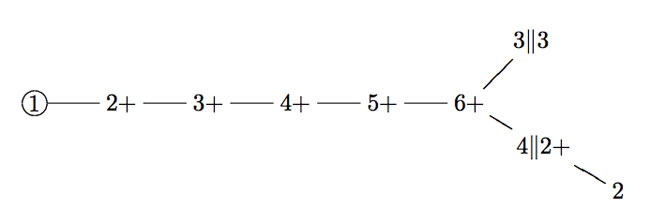Moreover, the dimensions of the groups give the exact dimension-components of the isotropic root of the extended E(8)-diagram. Further, the dimension of the group is equal to the order of the elements making up the conjugacy class of the monster to which exactly the given groups correspond via monstrous moonshine and hence compatible with John McKay‘s original E(8)-observation!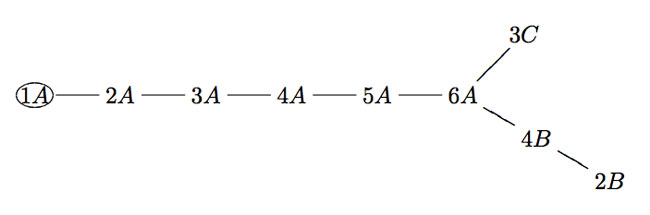Once again, I would love to hear when someone has more information on the cell-decomposition of the moonshine picture or if someone can extend the moonshine E(8)-graph, possibly to include all 171 moonshine groups.We have seen that Conway’s big picture helps us to determine all arithmetic subgroups of $PSL_2(\mathbb{R})$ commensurable with the modular group $PSL_2(\mathbb{Z})$, including all groups of monstrous moonshine.

As there are exactly 171 such moonshine groups, they are determined by a finite subgraph of Conway’s picture and we call the minimal such subgraph the moonshine picture. Clearly, we would like to determine its structure.

On the left a depiction of a very small part of it. It is the minimal subgraph of Conway’s picture needed to describe the 9 moonshine groups appearing in Duncan’s realization of McKay’s E(8)-observation. Here, only three primes are relevant : 2 (blue lines), 3 (reds) and 5 (green). All lattices are number-like (recall that $M \frac{g}{h}$ stands for the lattice $\langle M e_1 + \frac{g}{h} e_2, e_2 \rangle$).

We observe that a large part of this mini-moonshine picture consists of the three p-tree subgraphs (the blue, red and green tree starting at the 1-lattice $1 = \langle e_1,e_2 \rangle$. Whereas Conway’s big picture is the product over all p-trees with p running over all prime numbers, we observe that the mini-moonshine picture is a very small subgraph of the product of these three subtrees. In fact, there is just one 2-cell (the square 1,2,6,3).

Hence, it seems like a good idea to start our investigation of the full moonshine picture with the determination of the p-subtrees contained in it, and subsequently, worry about higher dimensional cells constructed from them. Surely it will be no major surprise that the prime numbers p that appear in the moonshine picture are exactly the prime divisors of the order of the monster group, that is p=2,3,5,7,11,13,17,19,23,29,31,41,47,59 or 71. Before we can try to determine these 15 p-trees, we need to know more about the 171 moonshine groups.

Recall that the proper way to view the modular subgroup $\Gamma_0(N)$ is as the subgroup fixing the two lattices $L_1$ and $L_N$, whence we will write $\Gamma_0(N)=\Gamma_0(N|1)$, and, by extension we will denote with $\Gamma_0(X|Y)$ the subgroup fixing the two lattices $L_X$ and $L_Y$.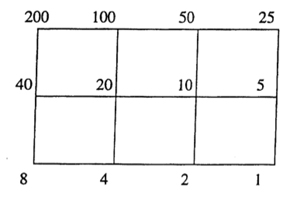As $\Gamma_0(N)$ fixes $L_1$ and $L_N$ it also fixes all lattices in the (N|1)-thread, that is all lattices occurring in a shortest path from $L_1$ to $L_N$ (on the left a picture of the (200|1)-thread).

If $N=p_1^{a_1} p_2^{a_2} \ldots p_k^{a_k}$, then the (N|1)-thread has $2^k$ involutions as symmetries, called the Atkin-Lehner involutions. For every exact divisor $e || N$ (that is, $e|N$ and $gcd(e,\frac{N}{e})=1$ we have an involution $W_e$ which acts by sending each point in the thread-cell corresponding to the prime divisors of $e$ to its antipodal cell-point and acts as the identity on the other prime-axes. For example, in the (200|1)-thread on the left, $W_8$ is the left-right reflexion, $W_{25}$ the top-bottom reflexion and $W_{200}$ the antipodal reflexion. The set of all exact divisors of N becomes the group $~(\mathbb{Z}/2\mathbb{Z})^k$ under the operation $e \ast f = \frac{e \times f}{gcd(e,f)^2}$.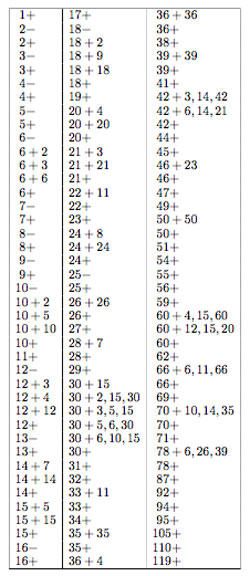Most of the moonshine groups are of the form $\Gamma_0(n|h)+e,f,g,…$ for some $N=h.n$ such that $h | 24$ and $h^2 | N$. The group $\Gamma_0(n|h)$ is then conjugate to the modular subgroup $\Gamma_0(\frac{n}{h})$ by the element $\begin{bmatrix} h & 0 \ 0 & 1 \end{bmatrix}$. With $\Gamma_0(n|h)+e,f,g,…$ we mean that the group $\Gamma_0(n|h)$ is extended with the involutions $W_e,W_f,W_g,…$. If we simply add all Atkin-Lehner involutions we write $\Gamma_0(n|h)+$ for the resulting group.

Finally, whenever $h \not= 1$ there is a subgroup $\Gamma_0(n||h)+e,f,g,…$ which is the kernel of a character $\lambda$ being trivial on $\Gamma_0(N)$ and on all involutions $W_e$ for which every prime dividing $e$ also divides $\frac{n}{h}$, evaluating to $e^{\frac{2\pi i}{h}}$ on all cosets containing $\begin{bmatrix} 1 & \frac{1}{h} \ 0 & 1 \end{bmatrix}$ and to $e^{\pm \frac{2 \pi i }{h}}$ for cosets containing $\begin{bmatrix} 1 & 0 \ n & 0 \end{bmatrix}$ (with a + sign if $\begin{bmatrix} 0 & -1 \ N & 0 \end{bmatrix}$ is present and a – sign otherwise). Btw. it is not evident at all that this is a character, but hard work shows it is!

Clearly there are heavy restrictions on the numbers that actually occur in moonshine. In the paper On the discrete groups of moonshine, John Conway, John McKay and Abdellah Sebbar characterized the 171 arithmetic subgroups of $PSL_2(\mathbb{R})$ occuring in monstrous moonshine as those of the form $G = \Gamma_0(n || h)+e,f,g,…$ which are

• (a) of genus zero, meaning that the quotient of the upper-half plane by the action of $G \subset PSL_2(\mathbb{R})$ by Moebius-transformations gives a Riemann surface of genus zero,
• (b) the quotient group $G/\Gamma_0(nh)$ is a group of exponent 2 (generated by some Atkin-Lehner involutions), and
• (c) every cusp can be mapped to $\infty$ by an element of $PSL_2(\mathbb{R})$ which conjugates the group to one containing $\Gamma_0(nh)$.

Now, if $\Gamma_0(n || h)+e,f,g,…$ is of genus zero, so is the larger group $\Gamma_0(n | h)+e,f,g,…$, which in turn, is conjugated to the group $\Gamma_0(\frac{n}{h})+e,f,g,…$. Therefore, we need a list of all groups of the form $\Gamma_0(\frac{n}{h})+e,f,g,…$ which are of genus zero. There are exactly 123 of them, listed on the right.

How does this help to determine the structure of the p-subtree of the moonshine picture for the fifteen monster-primes p? Look for the largest p-power $p^k$ such that $p^k+e,f,g…$ appears in the list. That is for p=2,3,5,7,11,13,17,19,23,29,31,41,47,59,71 these powers are resp. 5,3,2,2,1,1,1,1,1,1,1,1,1,1,1. Next, look for the largest p-power $p^l$ dividing 24 (that is, 3 for p=2, 1 for p=3 and 0 for all other primes). Then, these relevant moonshine groups contain the modular subgroup $\Gamma_0(p^{k+2l})$ and are contained in its normalizer in $PSL_2(\mathbb{R})$ which by the Atkin-Lehner theorem is precisely the group $\Gamma_0(p^{k+l}|p^l)+$.

Right, now the lattices fixed by $\Gamma_0(p^{k+2l})$ (and permuted by its normalizer), that is the lattices in our p-subtree, are those that form the $~(p^{k+2l}|1)$-snake in Conway-speak. That is, the lattices whose hyper-distance to the $~(p^{k+l}|p^l)$-thread divides 24. So for all primes larger than 2 or 3, the p-tree is just the $~(p^l|1)$-thread.

For p=3 the 3-tree is the (243|1)-snake having the (81|3)-thread as its spine. It contains the following lattices, all of which are number-like.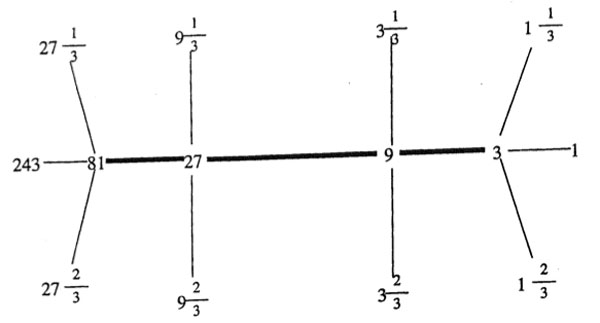Depicting the 2-tree, which is the (2048|1)-snake may take a bit longer… Perhaps someone should spend some time figuring out which cells of the product of these fifteen trees make up the moonshine picture!